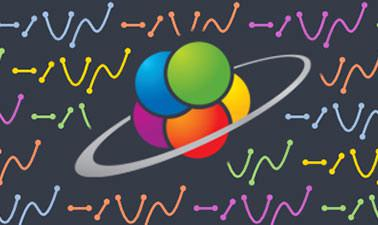Introduction to Algebra

Solve equations, draw graphs, and play with quadratics in this interactive course!There is one session available:

146,566 already enrolled! After a course session ends, it will be archivedOpens in a new tab.
Starts Jan 18
Ends Jul 1
Estimated 4 weeks
4–6 hours per week
Self-paced
Free

We live in a world of numbers. You see them every day: on clocks, in the stock market, in sports, and all over the news. Algebra is all about figuring out the numbers you don't see. You might know how fast you can throw a ball, but can you use this number to determine how far you can throw it? You might keep track of stock prices, but how can you figure out how much money you've made (or lost) in the market? And you may already know how to tell time, but can you calculate at what times a clock's hour and minute hands are exactly aligned? With algebra, you can answer all of these questions, using the numbers you already know to solve for the unknown. Algebra is an essential tool for all of high school and college-level math, science, and engineering. So if you're starting out in one of these fields and you haven't yet mastered algebra, then this is the course for you!

In this course, you'll be able to choose your own path within each lesson, and you can jump between lessons to quickly review earlier material. AlgebraX covers a standard curriculum in high school Algebra I, and CCSS (common core) alignment is indicated where applicable.

At a glance

• Language: English
• Video Transcript: English

What you'll learn

Skip What you'll learn
• How to add, subtract, multiply, and divide positive and negative integers, decimals, and fractions
• How to evaluate powers and roots, and simplify expressions with exponents
• How to solve single-variable and multi-variable equations and inequalities
• How to graph lines and inequalities, using both slope-intercept and point-slope form
• How to graph quadratics, and solve for their roots using the quadratic formula